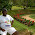## Thursday, October 20, 2016

### Geometry Problem 1274 Isosceles Triangle, 80-20-80 Degrees, Metric Relations, Measurement

Geometry Problem. Post your solution in the comment box below.
Level: Mathematics Education, High School, Honors Geometry, College.

This entry contributed by Sumith Peiris, Moratuwa, Sri Lanka.

Click on the figure below to view more details of problem 1274.1.Consider point D on segment BC such that AD=b. Tr. ACD is similar to Tr. ABC, we have CD=b^2/a
BD=a-(b^2/a)
In triangle BAD, Angle BAD=60. By cosine law a^2+b^2-ab =BD^2
We have
a^2+b^2-ab = [a-(b^2/a)]^2
On simplifying we get the desired result.
Trigonometric Solution.
sin(10)=b/2a,
sin (30)=1/2=3sin(10)-4sin(10)^3, replacing sin(10) by b/2a, we get the required expression.

1.If you let AD = b, then D = C, and you cannot form triangle ACD in your assumption.

2.In my opinion, Pradyumna has an excellent solution for this problem.
See below for the link to the sketch of his solution.

https://goo.gl/photos/oi63XrYgLS88ZzocA

Peter Tran

3.Thanks Peter for your kind words and diagram.

4.